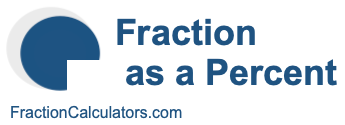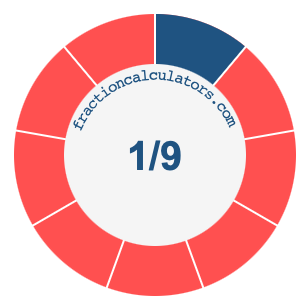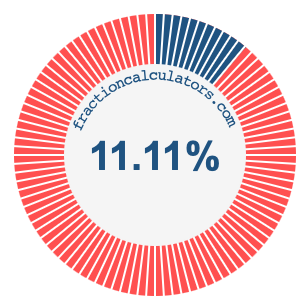1/9 as a percentWhat is 1/9 as a percent? Here we will explain and show you how to calculate and convert 1/9 to a percent.

In addition, we will also illustrate the fraction 1/9 on a pie chart and 1/9 as a percent on a pie chart.

1/9 is a fraction that means 1 out of 9, and percent means something out of 100. We can therefore make the following equation to get 1/9 as a percent:

 1 9
=
 percent 100

To find the percent in the equation above, we multiply 1 by 100 and then divide the product by 9. Here is the solution to the equation above and the answer to 1/9 as a percent:

 1 9
≈
 11.11 100

1/9 ≈ 11.11%

The illustrations below show you how 1/9 and 11.11% divide a pie chart differently, but cover the same area because they are the same.Fraction as a Percent Calculator
You can use this tool to convert another fraction to percent.

/

1/10 as a percent
Here is the next fraction on our list that we have converted to a percent for you.